1. Functions and Graphs

# Chapter 1 Review Exercises

True or False? Justify your answer with a proof or a counterexample.

1. A function is always one-to-one.

2.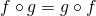, assuming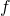and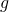are functions.

#### Solution

False

3. A relation that passes the horizontal and vertical line tests is a one-to-one function.

4. A relation passing the horizontal line test is a function.

#### Solution

False

For the following problems, state the domain and range of the given functions: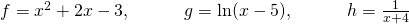5.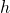6.#### Solution

Domain: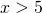, Range: all real numbers

7.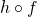8.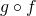#### Solution

Domain: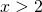or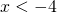, Range: all real numbers

Find the degree,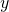-intercept, and zeros for the following polynomial functions.

9.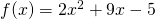10.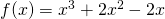#### Solution

Degree of 3,-intercept: 0, Zeros: 0,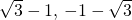Simplify the following trigonometric expressions.

11.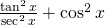12.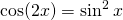#### Solution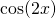or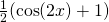Solve the following trigonometric equations on the interval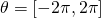exactly.

13.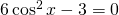14.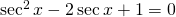#### Solution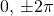Solve the following logarithmic equations.

15.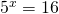16.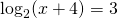#### Solution

4

Are the following functions one-to-one over their domain of existence? Does the function have an inverse? If so, find the inverse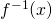of the function. Justify your answer.

17.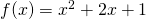18.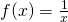#### Solution

One-to-one; yes, the function has an inverse; inverse: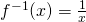For the following problems, determine the largest domain on which the function is one-to-one and find the inverse on that domain.

19.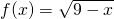20.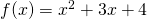#### Solution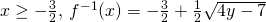21. A car is racing along a circular track with diameter of 1 mi. A trainer standing in the center of the circle marks his progress every 5 sec. After 5 sec, the trainer has to turn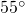to keep up with the car. How fast is the car traveling?

For the following problems, consider a restaurant owner who wants to sell T-shirts advertising his brand. He recalls that there is a fixed cost and variable cost, although he does not remember the values. He does know that the T-shirt printing company charges $440 for 20 shirts and$1000 for 100 shirts.

22. a. Find the equation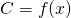that describes the total cost as a function of number of shirts and b. determine how many shirts he must sell to break even if he sells the shirts for $10 each. #### Solution a.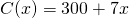b. 100 shirts 23. a. Find the inverse function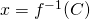and describe the meaning of this function. b. Determine how many shirts the owner can buy if he has$8000 to spend.

For the following problems, consider the population of Ocean City, New Jersey, which is cyclical by season.

24. The population can be modeled by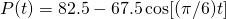, whereis time in months (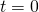represents January 1) and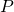is population (in thousands). During a year, in what intervals is the population less than 20,000? During what intervals is the population more than 140,000?

#### Solution

The population is less than 20,000 from December 8 through January 23 and more than 140,000 from May 29 through August 2

25. In reality, the overall population is most likely increasing or decreasing throughout each year. Let’s reformulate the model as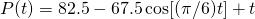, whereis time in months (represents January 1) andis population (in thousands). When is the first time the population reaches 200,000?

For the following problems, consider radioactive dating. A human skeleton is found in an archeological dig. Carbon dating is implemented to determine how old the skeleton is by using the equation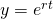, whereis the percentage of radiocarbon still present in the material,is the number of years passed, and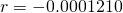is the decay rate of radiocarbon.

26. If the skeleton is expected to be 2000 years old, what percentage of radiocarbon should be present?

#### Solution

78.51%

27. Find the inverse of the carbon-dating equation. What does it mean? If there is 25% radiocarbon, how old is the skeleton?